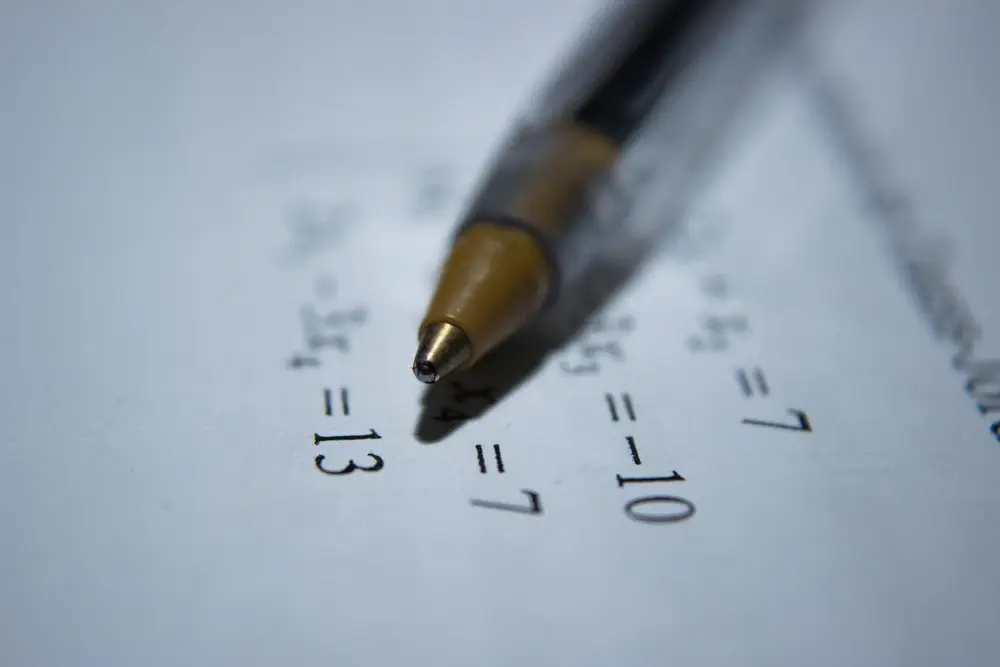# Sequences

A sequence of numbers is a string of values which all have a pattern from one number to the next. Sequences come in many forms and you need to know how to find patterns and also describe them.

An example of a sequence is:

2, 4, 6, 8, 10, 12, 14, 16, 18, 20, 22, etc.

In this sequence you add 2 to the previous number or multiply the position of the number by 2 (i.e. 1st number = 1 × 2 and the 10th number = 10 × 2).

Sequences can be defined in two ways:

An Inductive Definition
This describes the pattern using the previous number in the sequence. You need a number to start you off.
An example is: un+1 = un + 5 if u1 = 1
The sequence is - 1, 6, 11, 16, 21, 26, etc.

An Iteration
An iteration creates a sequence by using the position of the number. To find the first number you put 1 into the formula and to find the second number you put 2 into the formula.
An example is: un = 2n + 1
The sequence is - 3, 5, 7, 9, 11, 13, etc.

There are several types of sequences:

An arithmetic progression has an added fixed number
A geometric progression have terms multiplied by a fixed number
If a sequence gets closer to a number all the time it is convergent
If a sequence gets further away from a number is is divergent

To work out the nth term of a sequence, it is useful to use a table. Work out the difference between each value in your sequence. If the difference is always the same number e.g. 2,2,2,2 then the sequence is going to have 2n in it (it could be 2n+1 or something similar). If the difference is not the same e.g. 2,4,6,8 then there must be an x2 in the formulae.

Algebra

Algebra

Algebra

Algebra

Algebra

Algebra